Solution of Motion (Page No - 41) - Physics by Lakhmir Singh, Class 9

# Solution of Motion (Page No - 41) - Physics by Lakhmir Singh, Class 9 - Extra Documents & Tests for Class 9

Question 17:
Is the uniform circular motion accelerated ? Give reasons for your answer
Solution :
Yes, uniform circular motion is accelerated because the velocity changes due to continuous change in the direction of motion.

Question 18:
Write the formula to calculate the speed of a body moving along a circular path. Give the meaning of each symbol which occurs in it.
Solution :
The speed of a body moving along a circular path is given by the formula: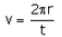where, v= speed

π=3.14 ( it is a constant)
r = radius of circular path
t= time taken for one round of circular path

Question 19:
Explain why, the motion of a body which is moving with constant speed in a circular path is said to be accelerated.
Solution :
The motion of a body which is moving with constant speed in a circular path is said to be accelerated because its velocity changes continuously due to the continuous change in the direction of motion.

Question 20:
What is the difference between uniform linear motion and uniform circular motion ? Explain with examples.
Solution :
Uniform linear motion is uniform motion along a linear path or a straight line. T he direction of motion is fixed. So, it is not accelerated. For e.g.: a car running with uniform speed of 10km/hr on a straight road.
Uniform circular motion is uniform motion along a circular path. T he direction of motion changes continuously. So, it is accelerated. For e.g.: motion of earth around the sun.

Question 21:
State an important characteristic of uniform circular motion. Name the force which brings about uniform circular motion.
Solution :
An important characteristic of uniform circular motion is that the direction of motion in it changes continuously with time, so it is accelerated.
Centripetal force brings about uniform circular motion.

Question 22:
Find the initial velocity of a car which is stopped in 10 seconds by applying brakes. The retardation due to brakes is 2.5 m/s2.
Solution :
Initial velocity, u=?
Final velocity, v=0m/s (car is stopped)
Retardation, a=-2.5 m/s2
Time, t=10s
v=u + at
0=u +(-2.5)x 10
u=25m/s

Question 23:
Describe the motion of a body which is accelerating at a constant rate of 10 m s2. If the body starts from rest, how much distance will it cover in 2 s ?
Solution :
The velocity of this body is increasing at a rate of ’10 metres per second’ every second.
Initial velocity, u=0m/s
Time, t=2s
Acceleration, a=10m/s2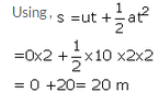Question 24:
A motorcycle moving with a speed of 5 m/s is subjected to an acceleration of 0.2 m/s2. Calculate the speed of the motorcycle after 10 seconds, and the distance travelled in this time.
Solution :
Initial velocity, u=5m/s
Final velocity, v=?
Acceleration, a=0.2m/s2
Time, t=10 sec
Using , v=u + at
v=5 + 0.2 x 10
v=5 + 2 =7 m/s
Now distance travelled in time is calculated;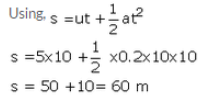Question 25:
A bus running at a speed of 18 km/h is stopped in 2.5 seconds by applying brakes. Calculate the retardation produced.
Solution :
Initial velocity, u=18km/h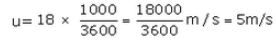Final velocity, v=0m/s
Time, t=2.5 sec
Acceleration, a=?
Using , v= u + at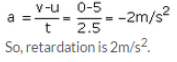Question 26:
A train starting from rest moves with a uniform acceleration of 0.2 m/s2 for 5 minutes. Calculate the speed acquired and the distance travelled in this time.
Solution :
Initial velocity, u=0m/s
Final velocity, v=?
Acceleration, a=0.2 m/s2
Time, t=5min= 5 x60=300 sec
Using, v = u + at
v =0 + 0.2 x 300=60m/s
And the distance travelled is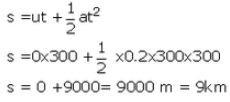Question 27:
Name the two quantities, the slope of whose graph gives :
(a) speed, and
(b) acceleration

Solution :
(a) Distance and Time
(b) Speed (or velocity) and Time

Question 28:
A cheetah starts from, rest, and accelerates at 2 m/s2 for 10 seconds. Calculate :
(a) the final velocity
(b) the distance travelled.

Solution :
Initial velocity,u=0 m/s
Final velocity, v=?
Acceleration, a=2 m/s2
Time, t=10s
(a)Using,
v = u + at
v=0 + 2 x 10= 20 m/s
(b)Distance travelled is: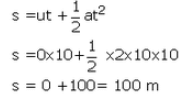Question 29:
A train travelling at 20 m s-1 accelerates at 0.5 m s-2 for 30 s. How far will it travel in this time ?
Solution :
Initial velocity, u=20m/s
Time, t=30 s
Acceleration,
a=0.5m/s2
Distance travelled is: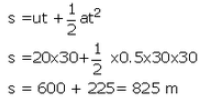Question 30:
A cyclist is travelling at 15 m s-1. She applies brakes so that she does not collide with a wall 18 m away. What deceleration must she have ?
Solution :
Initial velocity, u=15m/s
Final velocity, v=0m/s
Distance, s=18m
Acceleration, a=?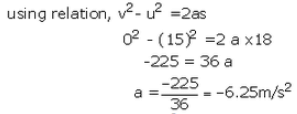So, deceleration is 6.25 m/s2.

Question 31:
Draw a velocity-time graph to show the following motion :
A car accelerates uniformly from rest for 5 s ; then it travels at a steady’ velocity for 5 s.
Solution :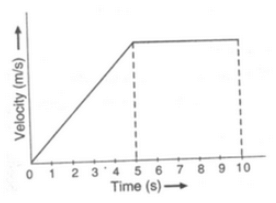Question 32:
The velocity-time graph for part of a train journey is a horizontal straight line. What does this tell you about
(a) the train’s velocity, and (b) about its acceleration ?

Solution :
(a) The train has a uniform velocity.
(b) There is no acceleration.

Question 33:
(a) Explain the meaning of the following equation of motion :v = u + at, where symbols have their usual meanings.
(b) A body starting from rest travels with uniform acceleration. If it travels 100 m in 5 s, what is the value of acceleration ?

Solution :
(a) v=u + at is the first equation of motion. It gives the velocity acquired by a body in time t when the body has initial velocity u and uniform acceleration a.
(b)Initial velocity, u=0m/s
Time, t=5 s
Distance, s=100m
Acceleration, a=?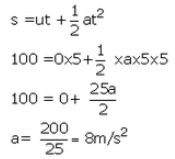Question 34:
(a) Derive the formula : v = u + at, where the symbols have usual meanings.
(b) A bus was moving with a speed of 54 km/h. On applying brakes it stopped in 8 seconds. Calculate the acceleration.
Solution :
(a) Consider a body having initial velocity ‘u’. Suppose it is subjected to a uniform acceleration ‘a’ so that after time’t’ its final velocity becomes ‘v’. Now, from the definition of acceleration we know that:
(b) Initial velocity, u=54km/h= 15m/s
Final velocity, v=0m/s
Time, t=8s
Acceleration, a=?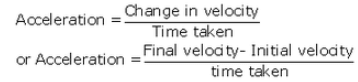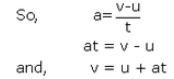where v= final velocity of the body

u = initial velocity of the body

a = acceleration

and t= time taken

(b) initial velocity , u = 54 km/h = 15m/s

Final velocity, v = 0 m/s

Time, t = 8s

Acceleration a= ?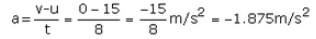Question 35:
(a) Derive the formula :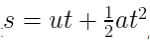, where the symbols have usual meanings.
(b) A train starting from stationary position and moving with uniform acceleration attains a speed of 36 km per hour in 10 minutes. Find its acceleration.
Solution :
(a) Suppose a body has an initial velocity ‘u’ and a uniform acceleration’ a’ for time ‘t’ so that its final velocity becomes ‘v’. Let the distance travelled by the body in this time be ‘s’. The distance travelled by a moving body in time ‘t’ can be found out by considering its average velocity. Since the initial velocity of the body is ‘u’ and its final velocity is ‘v’, the average velocity is given by:
(b) Initial velocity, u=0m/s

Final velocity, v=36km/h=10m/s
Time, t=10min =10 x 60=600 sec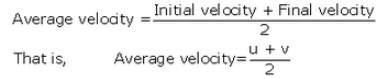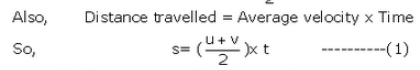From the first equation of motion, we have v= u+at

Put the value of v in equation (1), we get: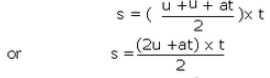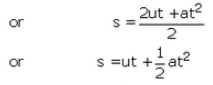Where s = distance travelled by the body in time t

u = initial velocity of the body

and a = acceleration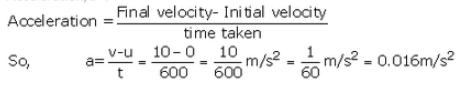Question 36:
(a) Write the three equations of uniformly accelerated motion. Give the meaning of each symbol which occurs in them.
(b) A car acquires a velocity of 72 km per hour in 10 seconds starting from rest. Find
(i) the acceleration,
(ii) the average velocity, and
(iii) the distance travelled in this time.
Solution :

(b) Initial Velocity , u = 0 m/s

Final velocity, v = 72km/h = 20m/s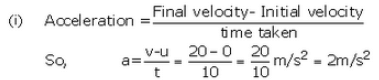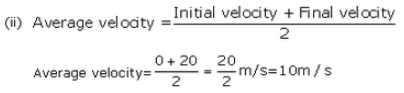(iii) Distance travelled = Average velocity x time

= 10 m/s  x 10 sec = 100m

Question 37:
(a) What is meant by uniform circular motion ? Give two examples of uniform circular motion.
(b) The tip of seconds’ hand of a dock takes 60 seconds to move once on the circular dial of the clock. If the radius of the dial of the clock be 10.5 cm, calculate the speed of the tip of the seconds’ hand of the clock. (Given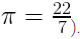Solution :
(a) When a body moves in a circular path with uniform speed (constant speed), its motion is called uniform circular motion. For e.g.
(i) Artificial satellites move in uniform circular motion around the earth.
(ii) Motion of a cyclist on a circular track.
(b) The speed of a body moving along a circular path is given by the formula: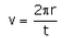Given, t=60 sec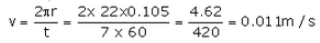The document Solution of Motion (Page No - 41) - Physics by Lakhmir Singh, Class 9 | Extra Documents & Tests for Class 9 is a part of the Class 9 Course Extra Documents & Tests for Class 9.
All you need of Class 9 at this link: Class 9

## Extra Documents & Tests for Class 9

1 videos|228 docs|21 tests

## FAQs on Solution of Motion (Page No - 41) - Physics by Lakhmir Singh, Class 9 - Extra Documents & Tests for Class 9

 1. What is motion in physics?Ans. Motion in physics refers to the change in position of an object with respect to its surroundings over time. It involves the concept of distance, displacement, speed, velocity, and acceleration.
 2. What are the different types of motion?Ans. There are various types of motion in physics, including linear motion, circular motion, periodic motion, random motion, and projectile motion.
 3. How is speed different from velocity?Ans. Speed is a scalar quantity that measures the rate of change of distance, whereas velocity is a vector quantity that measures the rate of change of displacement. Velocity includes both the magnitude (speed) and direction of motion.
 4. What is the equation to calculate average speed?Ans. The equation to calculate average speed is: Average Speed = Total Distance Traveled / Total Time Taken. It gives the overall rate of motion of an object over a given time period.
 5. How does acceleration affect motion?Ans. Acceleration is the rate of change of velocity. If an object experiences acceleration, its velocity will change over time. An object can either accelerate or decelerate depending on whether the acceleration is in the same direction as its velocity or in the opposite direction.

## Extra Documents & Tests for Class 9

1 videos|228 docs|21 testsExplore Courses for Class 9 examSignup to see your scores go up within 7 days! Learn & Practice with 1000+ FREE Notes, Videos & Tests.
10M+ students study on EduRev
Track your progress, build streaks, highlight & save important lessons and more!
Related Searches

,

,

,

,

,

,

,

,

,

,

,

,

,

,

,

,

,

,

,

,

,

,

,

,

;